Ch 7. Incompressible and Inviscid Flow Multimedia Engineering Fluids Bernoulli'sEquation FlowMeasurements Superpositionof Flows Flow aroundCylinder
 Chapter 1. Basics 2. Fluid Statics 3. Kinematics 4. Laws (Integral) 5. Laws (Diff.) 6. Modeling/Similitude 7. Inviscid 8. Viscous 9. External Flow 10. Open-Channel Appendix Basic Math Units Basic Equations Water/Air Tables Sections Search eBooks Dynamics Fluids Math Mechanics Statics Thermodynamics Author(s): Chean Chin Ngo Kurt Gramoll ©Kurt GramollFLUID MECHANICS - THEORY In this section, the solutions for potential flow past a fixed and rotating circular cylinder will be obtained by the method of superposition. Flow Past a Fixed Circular Cylinder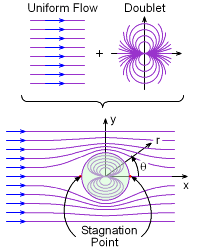Superposition of a Uniform Flow and a Doublet Gives Flow over a Cylinder Flow past a fixed circular cylinder can be obtained by combining uniform flow with a doublet. The superimposed stream function and velocity potential are given by      Ψ = Ψuniform flow + Ψdoublet = U r sinθ - K sinθ/r and      Φ = Φuniform flow + Φdoublet = U r cosθ + K cosθ/r respectively. Since the streamline that passes through the stagnation point has a value of zero, the stream function on the surface of the cylinder of radius a is then given by      Ψ = U a sinθ - K sinθ/a = 0 which gives the strength of the doublet as      K = U a2 The stream function and velocity potential for flow past a fixed circular cylinder become      Ψ = Ur [1 - (a/r)2] sinθ and      Φ = Ur [1 + (a/r)2] cosθ respectively. The plot of the streamlines is shown in the figure. The velocity components can be determined by: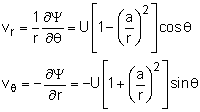Along the cylinder (r = a), the velocity components reduce to      vr = 0     and     vθ = -2U sinθ The radial velocity component is always zero along the cylinder while the tangential velocity component varies from 0 at the stagnation point (θ = π) to a maximum velocity of 2U at the top and bottom of the cylinder (θ = π/2 or -π/2). The pressure distribution along the cylinder can be obtained using Bernoulli's equation,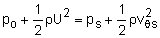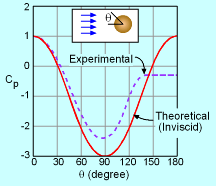Comparison between Experimental and Theoretical Potential Flow Results where the subscript "o" refers to the upstream condition while the subscript "s" refers to the condition along the cylinder. The elevation changes are assumed negligible. Substituting the expression for vθs into the above equation and rearranging gives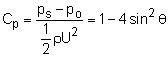where Cp is the dimensionless pressure coefficient. The plot of Cp as a function of θ is shown in the figure. The discrepancy between the experimental and theoretical results, as shown in the figure, is due to the viscous effects.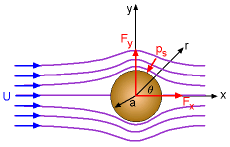Drag and Lift The concepts of drag and lift will be briefly introduced here. The drag developed on the cylinder can be obtained by integrating the pressure over the cylinder surface as,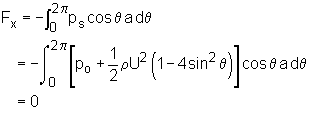Drag is the resultant force exerted by the fluid on the cylinder, and its direction is parallel to the upstream uniform flow direction. The lift is the resultant force acting perpendicular to the uniform flow direction, and it can be obtained by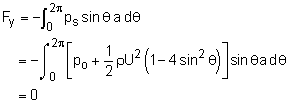When the integrations are carried out for Fx and Fy (integration details are not given for simplicity), it is found that both drag and lift are zero for potential flow. The potential flow solutions developed in this section are based on the assumption of inviscid flow (i.e., zero viscosity), which implies that drag vanishes. However, as will be discussed in the Lift section, when a real fluid flows past a cylinder, viscous effects are important near the cylinder. Viscous effects will cause the flow to separate away from the cylinder, and the drag is nonzero in actual flow situations. This discrepancy is referred to as d'Alembert's paradox.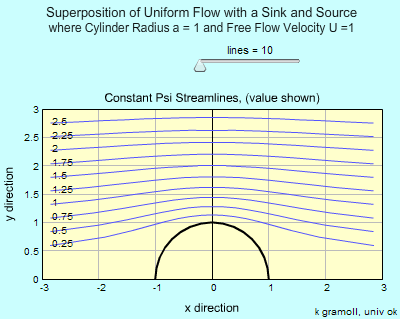Combination of Uniform Flow and Doublet Flow Past a Rotating Circular Cylinder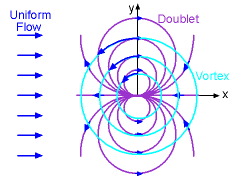Superposition of a Uniform Flow, a Doublet and a Vortex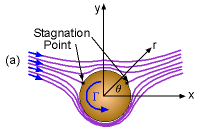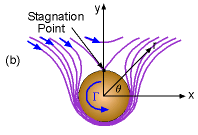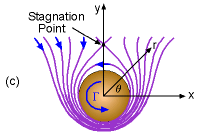Streamlines of Flow Past a Rotating Cylinder where    (a) Γ / (4πaU) <1, (b) Γ / (4πaU)=1 and (c) Γ / (4πaU) >1 When the solutions obtained previously for flow past a fixed cylinder are combined with a vortex, flow past a rotating cylinder can be simulated. The superimposed stream function and velocity potential now consist of three components and are given by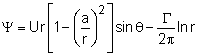and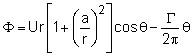where Γ is the strength of the vortex circulation. The radial velocity vr is still zero along the cylinder surface while the tangential velocity is given by,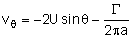From the above equation, it can be found that the location of the stagnation point, θstag, depends on the strength of the circulation. Setting vθ equal to zero and yields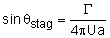The streamlines along with the location of the stagnation point for different dimensionless circulation strengths ( Γ / [2πaU] ) are shown in the figure. The pressure distribution along the cylinder surface can be obtained by Bernoulli's equation, giving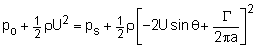This is generally rearranged in terms of the dimensionless pressure coefficient, Cp,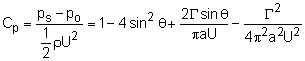The drag and lift are obtained by integrating the pressure over the cylinder surface, giving      Fx = 0      and      Fy = -ρUΓ Hence, there is still no drag for a rotating cylinder (inviscid assumption). However, there is lift involved, and it is directly proportional to the density, upstream velocity and strength of the vortex (Kutta-Joukowski law). The lifting effect for rotating bodies in a free stream is called the Magnus effect.

Practice Homework and Test problems now available in the 'Eng Fluids' mobile app
Includes over 250 problems with complete detailed solutions.
Available now at the Google Play Store and Apple App Store.